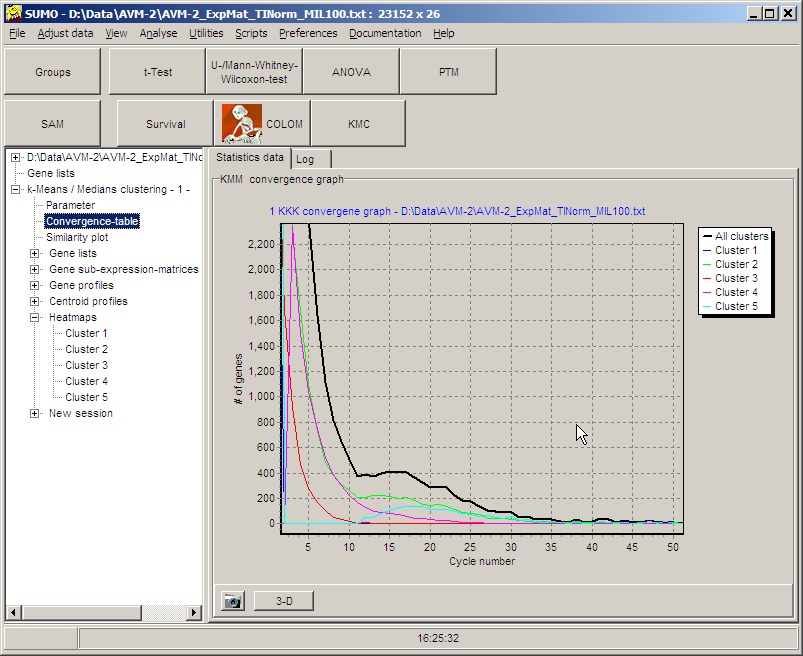# K-means / -medians Clustering

Cluster analysis is one method of non-supervised machine-learning.

Main objectives are:

• Assignment of objectives to classes is unknown, i.e. there is no dependant variable
• All objects of a partition  ( a class) should be similar.
• There is no a priori knowledge, which object belongs to which class => the assignment of objects to classes can not be validated

Clustering is a heuristic method.
Many clustering methods are initialized with randomized start values, with the result that different runs may result in different clusters.
Often, a clustering method is repeatedly applied to the same dataset. A human expert then validates quality and meaning of the clusters and selects optimal classification.

K-Means Clustering is a iterative distance based clustering method. In opposite to totally non-supervised hierarchical clustering, k-means clustering requires one parameter: K, the number of clusters to search for.
The goal is to divide the objects (genes in the expression matrix) into a user defined number of K clusters in such a way that all members of the cluster have the same common pattern, different to all other clusters.

The user has to define the number of clusters K for which the algorithm shall try to build cluster for.
Often the number of clusters may be guessed according to a hypothesis. But in most cases it is hard to determine the correct number of clusters, even on well known problems:
Define number of clusters for playing cards

• Red / Black => 2 clusters, Clubs,
• Spades, Hearts, Diamonds => 4 clusters,
• ...

Not depending of the internal structure of the data, K-means clustering will always try to dissect the data into the requested number of k clusters. Independent of the real internal data structure.

• a too high number of clusters, redundant clusters may be created,
• too small number of clusters, sub-classes may be lost

### Basic algorithm:

i.    Define number of clusters to search for
ii.    (Randomly) assign cluster centroids

1)    Calculate distance of each gene to each cluster centroid
2)    Re-assign genes to that cluster where distance is minimal
3)    Calculate cluster centroids (means)
4)    Continue at step 1 until no genes move any longer between clusters, a max number of cycles is exceeded, ...

Imagine a dataset which shall be divided into two clusters:ii. Randomly assign centroids to the two clusters: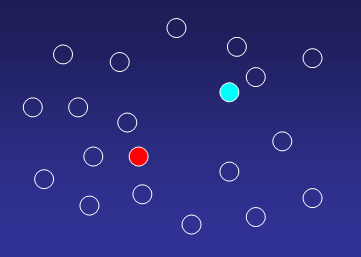Compute distances from each gene to all cluster centroids, and assigne a gene to that cluster where distance to centroid is minimal: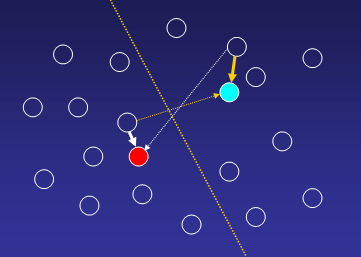1. Until all genes are uniquely assign to one of the k-clusters: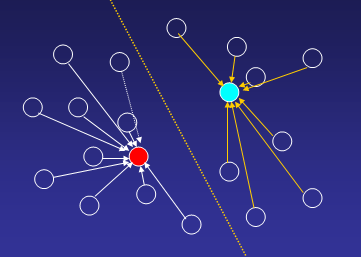2. Re-compute cluster centroids with new cluster members: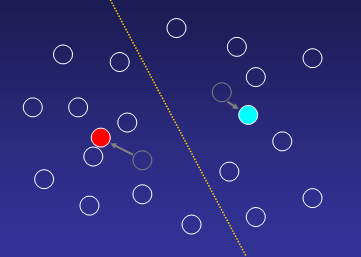3. Reassign genes to new cluster centroids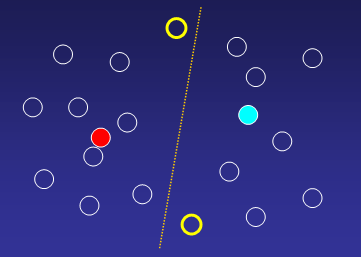### Parameters

Having selected KMC by clicking the KMC-button ( or from Main menu | Analyse | KMC), the Group Selection / Parameter dialog pops up.

ON the Groups tabsheet, the hybridisations used for a KMC analyses may be selected: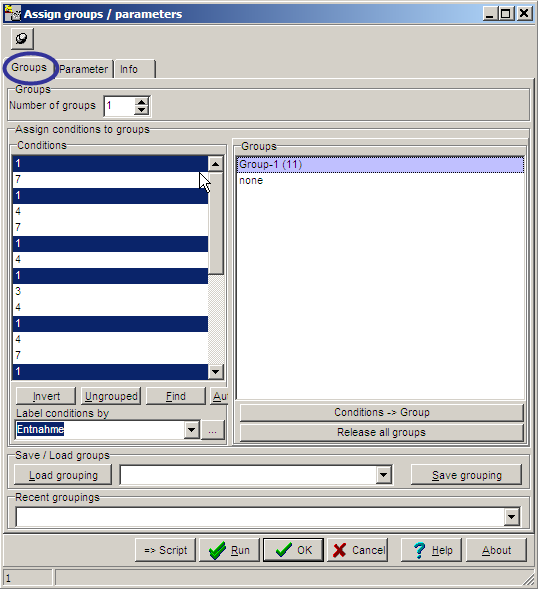On the Parameters tab sheet, basic computation parameters may be set: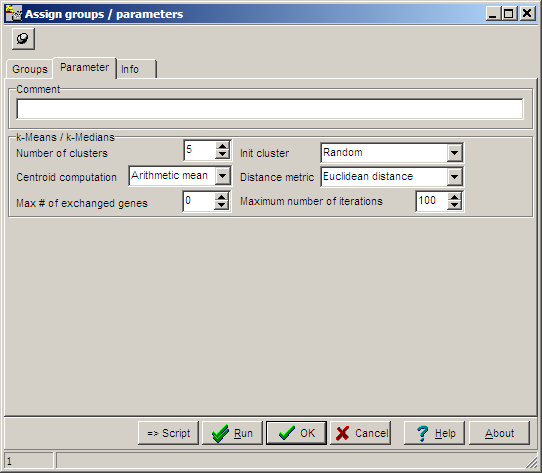Number of clusters K, the cluster number, default value=5 Init cluster Method how to initialize cluster centroids:  - Random, random values are assign to the centroids  - Regular wave pattern Centroid computation The method how to compute cluster centroids from member genes:  - Arithmetic mean  - Median Distance metric The method how to compute Centroid <=> Gene distances:  - Euclidean distance  - cosine of angle between genes vectors (~ Pearson correlation)  - Dot product Max # of exchanged genes Stop criterion1: KMC count the total number of genes exchanged between all clusters. As soon as this number is small enough, KMC stops. Maximum number of iterations Stop criterion 2: Maximumn number of cycles "Assign genes/Re-compute centroids". Useful in cases where KMC does not converge: i.e. there is no preferable gene=>cluster assignment.

### Data views

After the analyses a new branch is added to the Analysis tree: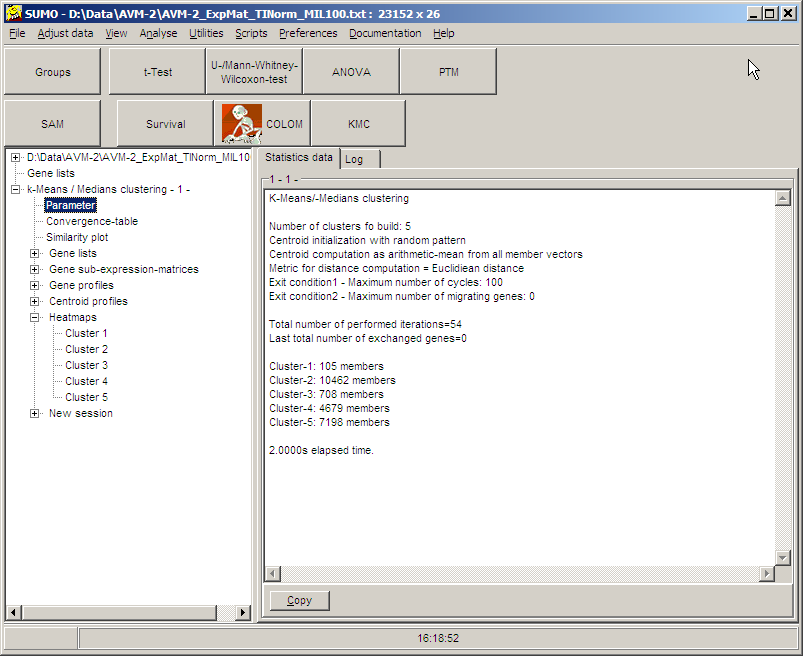As usual, Gene lists, Sub-expression-matrices, Gene-/Centroid profiles or heat maps for the respective cluster may be displayed, by clicking the respective tree item.

### Convergence table:

The convergence table displays graphically how fast each single cluster  as well as the whole KMC-analyses converges to its final classification: